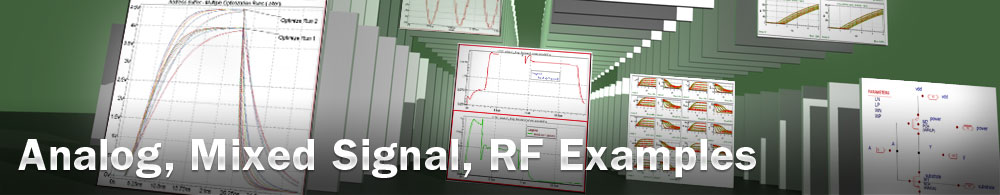Resistor Model Extraction

## opt_ex13 : Resistor Model Extraction

Requires: Utmost IV, SmartSpice, SmartView

Minimum Versions: Utmost IV 1.10.6.R, SmartSpice 4.10.2.R, SmartView 2.28.2.R

This example describes how to extract a resistor model including temperature coefficients and delta geometry parameters.

The project file opt_ex13.prj and the data file opt_ex13.uds for this example should both be loaded into your database. When opened, the project will look as shown in .

The optimization sequence, which fully automates the extraction of this resistor model example, has three sections. The objective of each section is to isolate a device characteristic and then to extract or optimize only those model parameters which account for this device behavior.

The first section, selects a single resistor measurement at the nominal temperature. We chose the largest measured resistor to minimize the effects of delta width and delta length as shown in . From this single dataset, we can extract the sheet resistance assuming that delta geometry effects are zero. This is done by the extraction 'rsh' which is defined as shown in . The extraction setup, also called 'rsh', is used to select the extraction to be performed on the single dataset and also defines where to write the result as shown in . In this case, the extraction called 'rsh' is performed and the result is written into the model parameter RSH of the model RES in the optimized column.

The second section deals with the delta geometry parameters. These are extracted from all of the available resistor geometries measured at the nominal temperature. The dataset subset definition is as shown in . In order to optimize the delta width parameter DW , you must have at least two resistors with different widths. Likewise, you need at least two different lengths to extract the delta length parameter DLR . In this example, there are resistors with three different widths with the same length and three different lengths with the same width.

The previous section extracted a preliminary value for the RSH parameter assuming that the delta width and delta length are both zero. This section optimizes all three parameters RSH, DW, DLR as shown in . Once the nominal temperature model has been extracted, the measured versus simulated data will be displayed in the viewer as shown in .

The final section deals with the temperature coefficients. One resistor has been measured at five temperatures and the dataset subset definition is as shown in . In the optimization setup shown in , there are two targets defined. The first is the measured IV chacteristic for the resistors, the second is a plot of extracted resistance value 'res' vs the temperature. Both of these targets will be used during the optimization. The first and second order temperature coefficients TC1 and TC2 are optimized. Once this optimization has been run, the measured vs simulated IV and extracted characteristics are displayed in the viewer as shown in .

The results from the extraction and the optmizations are also shown in the project status log area as shown in . The model card can then be exported into an external model library file as shown in the output file opt_ex13.lib.